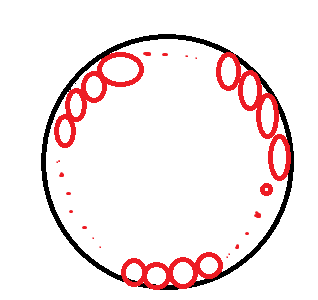# A lovely one

Geometry Level 4If , inside a big circle , exacty 36 small circles , each of radius 5 , can be drawn in such a way that each small circle touches the big circle and also touches both its adjacent small circles , then the radius of the big circle is a

Find $[ a]$

Clarification -

• The image is just shown to understand the given situation , here all the small circles are of same radius and each one touches its adjacent one and the circle in which it is inscribed.

• [.] - Greatest integer function

Note - If you can give a general formula for the radius of the big circle such the there are more than or equal to 3 small circles inscribed in it , you will be appreciated

×